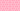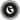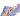PricingResources

# What Is Z-Score?Written by

You see a promising investment opportunity, but you want assurance that the company isn’t going to go belly-up with your money. This is where a z-score comes in handy. Also known as a standard score, New York University professor Edward Altman developed the z-score in the late 1960s. Altman’s goal was to determine the likelihood of bankruptcy of publicly traded companies. In addition to being a credit-strength test, z-scores are now used by traders to predict market volatility and by investors assessing the financial health of businesses.

## Z-score definition

Technically speaking, a z-score is a statistical measurement that shows a value's relationship to the mean. Z-scores indicate if a value is typical or atypical for a data set. They also make it possible for scores to be adapted from various data sets so that they can be compared to one another more accurately.

In the financial sector, a z-score of 1.8 or lower indicates that a company may be headed for bankruptcy and, as such, potentially represents an unwise investment. A score of 3 or higher indicates financial stability, and that the company has the potential to be a solid investment choice.

## Z-score vs. standard deviation: what’s the difference?

Standard deviation is a calculation of the amount of variability within a specified set of data. Z-score, however, represents the number of standard deviations a specified data point lies from the mean.

## How to calculate z-score

Z-scores can be calculated by hand, using Excel, or with the aid of a graphing calculator. You can also use a z-score calculator online.

To calculate a basic z-score, you need to know the mean (μ), the population standard deviation (σ) and the value being compared to the mean (x), also known as the raw or test score. The basic z-score formula is:

In the financial sector, the z-score formula is more complex as it’s based on five key financial ratios. This information can be found in a company’s financial accounting documents and on the Annual Return (AR1). The calculation used to determine the z-score from this information is:

ζ = 1.2A + 1.4B + 3.3C + 0.6D + 1.0E

where:

Zeta (ζ)  = The Altman Z-score

A = Working capital / total assets

B = Retained earnings / total assets

C = Earnings before interest and taxes (EBIT) / total assets

D = Market value of equity / book value of total liabilities

E = Sales / total assets

The Z-score table

Standard scores are represented visually in graphs and tables. In graph format, z-scores can be plotted on a normal distribution curve. A z-score table, also called a standard normal table, shows the percentage of values above (to the right) or below (to the left) a z score on a standard normal distribution.

If your z-scores are positive, you’ll use the positive z-score table that shows the values to the right of the mean. And accordingly, a negative table is used for negative z-scores scores appearing to the left of the mean. The type of table you choose will depend on the data derived from your calculations and the financial trends it reveals.

A z-score table displays the relationship between the data in a visual format and supports the analysis and decision-making process. Analysing z-score examples can give investors and business owners an idea of their utility, function, as well as how to calculate z-score for financial planning and investment.

#### We can help

GoCardless helps you automate payment collection, cutting down on the amount of admin your team needs to deal with when chasing invoices. Find out how GoCardless can help you with ad hoc payments or recurring payments.Contact sales

Sales

Support